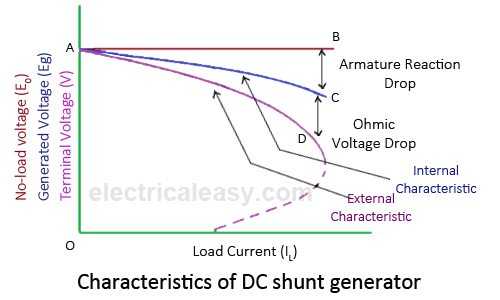# Test: Operating Characteristics of DC Generators

## 10 Questions MCQ Test Electrical Machines | Test: Operating Characteristics of DC Generators

Description
Attempt Test: Operating Characteristics of DC Generators | 10 questions in 10 minutes | Mock test for Electrical Engineering (EE) preparation | Free important questions MCQ to study Electrical Machines for Electrical Engineering (EE) Exam | Download free PDF with solutions
QUESTION: 1

### Which of the following characteristics reveal about the magnetization nature of the machine?

Solution:

Both no-load as well as load characteristics are required to predict the magnetization of the machine.

QUESTION: 2

### Choose the most inappropriate out of the following for the no-load characteristics of the dc generator.

Solution:

All the mentioned nature of the characteristics are correct.

QUESTION: 3

### The external characteristic is plotted between

Solution:

The external characteristic is plotted between terminal voltage and armature current at fixed excitation. ... Explanation: Armature characteristic is also known as regulation characteristic. It is so called due to the fact that the difference in the terminal voltage helps to determine the voltage deviation.QUESTION: 4

A student forgot to mark the x-y axes in his experiments but he just noted down the cause and the effect for each. How will he conclude about the armaturecharacteristic out of the all plotted graphs?

Solution:

Armature characteristic is observed at constant terminal voltage for emf vs armature current.

QUESTION: 5

Armature characteristic is also known as

Solution:

Armature characteristic is also known as regulation characteristic. It is so called due to the fact that the difference in the terminal voltage helps to determine the voltage deviation.

QUESTION: 6

The air gap line represents

Solution:

Air gap line in the magnetization curve represents the ideal nature of the machine considering no saturation for the dc machine.

QUESTION: 7

For a given dc generator, the external characteristic is plotted . Without using further plots, how can we obtain internal characteristic?

Solution:

Adding the armature resistance drop we can obtain the external characteristic for a dc generator.

QUESTION: 8

The voltage drop in terminal voltage from no-load to full load in a shunt generator can be compenated using

Solution:

By aiding the series field the armature reaction drop can be compensated.

QUESTION: 9

Why is armature of a dc machine made of silicon steel stampings?

Solution:

The silicon steel has very high permeability and makes the flux past consistent. Thereby making lesser losses.

QUESTION: 10

Armature reaction in a generator results in

Solution:Use Code STAYHOME200 and get INR 200 additional OFF Use Coupon Code